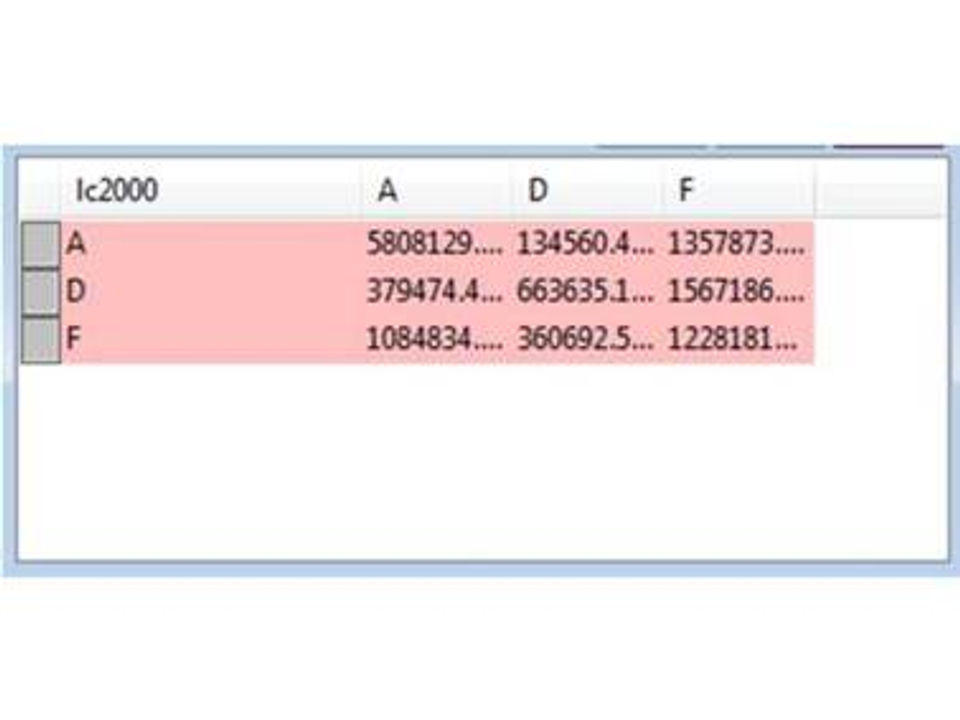top of page
Search

# ARC/INFO Functions in SQL – Tabulate Areas

I love the Tabulate Area command in ArcGIS.  The ability to create a cross tabulation of two feature datasets is very cool.  SQL has cross tabulation commands (heck, even Excel does this through a pivot table), and because we are saying that spatial data is just another datatype, we can leverage the cross tabulation queries in SQL to work on, say a spatial relationship like land use values from two different years, instead of looking at sales by store.

Lets assume we have land use values from two different years: 1970 and 2000 (I use this example with my students every year).  We will also assume that there are two fields called LC1970 and LC2000.  An example map looks like the following:The SQL code to generate this is actually standard use of the TRANSFORM command with a PIVOT command.  This is standard SQL stuff – the only spatial thing is that we are creating a table of the intersection between the 1970 and 2000 land use categories.

TRANSFORM SUM(Area(g)) SELECT lc2000 FROM    (SELECT ClipIntersect([lu1970].[Geom (I)],[lu2000].[Geom (I)]) AS g, [lc1970],[LC2000]    FROM [lu1970],[lu2000]    ) WHERE lc1970 <> “” and lc2000 <> “” GROUP BY lc2000 PIVOT lc1970

The ClipIntersect is a spatial SQL construct that performs a geometric intersection of the two layers on-the-fly, in memory.  BTW, this is another benefit of SQL – there are no orphaned files lying around – most of it is done in memory and then released.

The resulting table from the example data set looks like this: